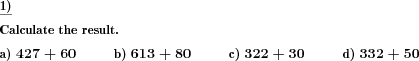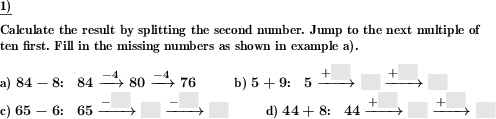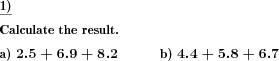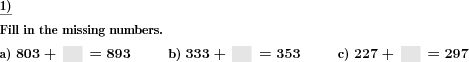Custom math worksheets at your fingertips# Details for problem "Add multiples of powers of ten"

Quickname: 8201

Elementary School, Primary School, Middle School.

## Summary

Multiples of powers of ten added to natural numbers.

## Example## Description

Give a boost to basic addition skills by using these worksheets to add multiples of powers of ten without any carries.

In this addition problem, the first summand is a natural positive number with a defined number of places. The second summand is a multiple of a power of ten.

That means that the second summand's first digit will be in the range 1-9, while the other ones will be zero. The second number will always have fewer places than the first one. Carries will never occur.

The complexity of problems can be selected as per grade level from second-grade to fifth-grade, to be used as a "do now" bell-ringer activity, anticipatory set, and closure exercise. This math worksheet would also be perfect for a quick assessment tool.

By completing this worksheet successfully, the following milestones can be achieved.

1. The addition of numbers will be revised.
2. In-depth understanding of adding a digit to 0.
3. Strong concepts of the addition of multiple digit numbers will be achieved through practice.
4. The practice of these worksheets will also lay the foundation for the concept of additive identity.

In each problem, two positive numbers are given to be added without carries. Following customized options are available.

• The number of problems can be selected from 1 to 10.
• The number of digits in both numbers can be selected in a range between 1 to 6.
• The number of 0 in the rightmost place can also be selected.

The answer key will provide the sum of 2 numbers for all problems.

Download free printable worksheets for this math problem here. The worksheet contains the problems only, the solution sheet includes the answers. Just click on the respective link.

•Worksheet 1Solution sheet with answers
•Worksheet 2Solution sheet with answers
•Worksheet 3Solution sheet with answers

If you can not see the solution sheets for download, they may be filtered out by an ad blocker that you may have installed. If this is the case, please allow ads for this page and reload the page. The solution sheets will then reappear.

• Do these sample worksheets do not really fit?
• Do you need more math worksheets, with a different level of difficulty?
• Would you like to combine different problems on a worksheet and adjust them to your needs?
• As a teacher, you can put together your own worksheets using the automatically generated math problems provided.
With a free initial credit, you can start creating your own math worksheets in a few minutes.

You can try it for free! Register here, to create custom worksheets now!

## Customization options for this problem

Parameter
Possible values
Number of problems
1, 2, 3, 4, 5, 6, 7, 8, 9, 10
First number places
1, 2, 3, 4, 5, 6
Second number places
1, 2, 3, 4, 5, 6
Rightmost places 1st number>0
Yes, No

## Similar problems

Remark
Description
With blanks to be filled in
What multiple of a power of ten has been added?

## Other types of problems that appear on worksheets with this problem:

Relevance
Name
Description
Quickname
Example
****
Add and subtract spliting one digit number
Addition and subtraction by splitting one digit number****
Numbers with or without decimal places have to be added.****
Addition of powers of ten, fill blanks
What multiple of a power of ten has been added?****
Determine shapes with same shaded fraction
In a series of shapes find the two shapes that represent the same fraction.